## PIGGY BANK REVISITED

### SUBTRACT DECIMALS AND ADD NEGATIVE INTEGERS

Understand the ralationship between fractions and decimals, subtracting decimals, and adding negative integers.

## Intentionality & Unit Overview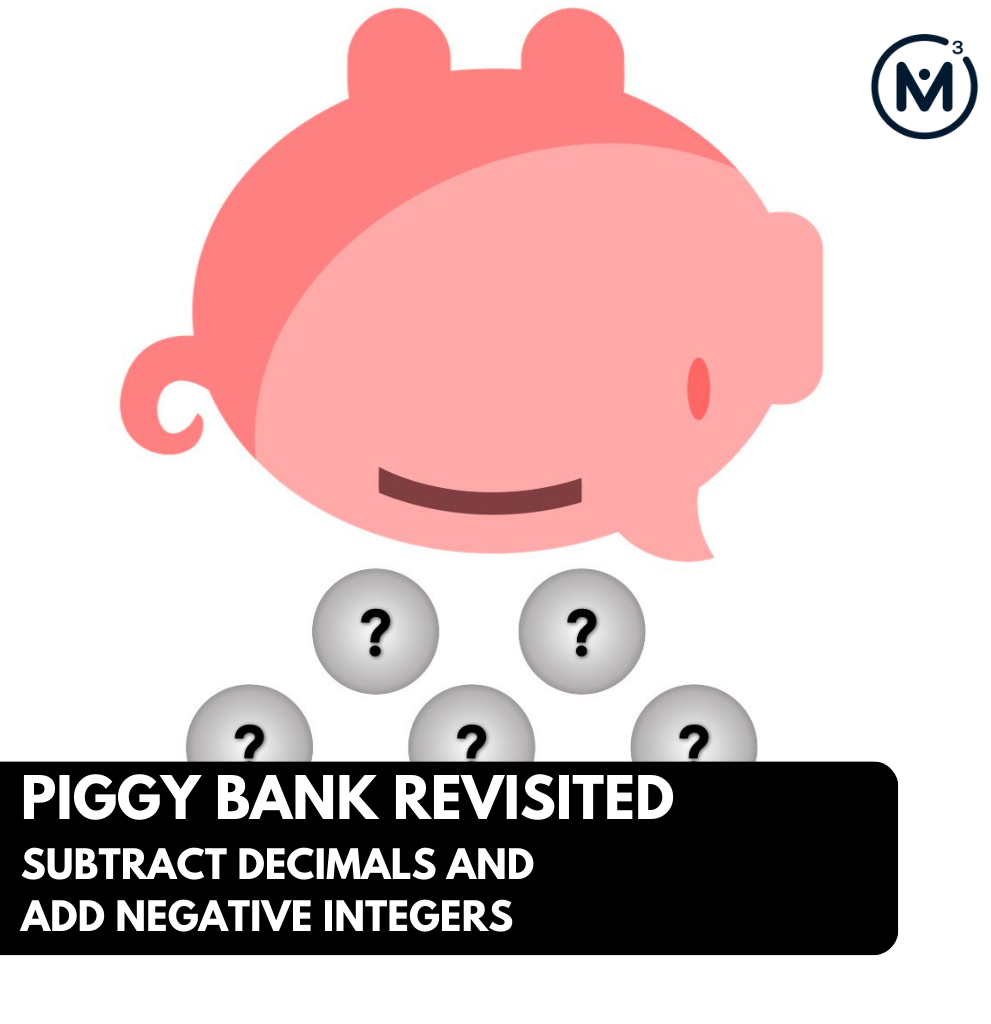### Access each lesson from this unit using the navigation links below

Consider the initial value of coins in the piggy bank, and the remaining value after coins have been removed. Based on the remaining value of money in the piggy bank, predict the value of each coin that was removed through this missing subtrahend scenario. Represent the context using a model and as a single equation.

## Intentionality…

In this task, students explore the relationship between fractions and decimals. In particular, we will explore the equivalence between coins, expressed as fractions of a whole dollar, and their corresponding decimal representation.

The purpose of this lesson is to encourage students to think flexibly about these two representations. When operating with fractions and decimals, at times, it will be more convenient to think of the fraction as a decimal, and vice versa. In today’s task, students will tackle a missing subtrahend that may elicit a linear model to determine the difference between the initial value and the remaining value. The context expressed as an equation will create an opportunity to explore the use of variables.

All tasks within this unit are intended to be completed without the use of a calculator to support reasoning and thinking.

Some of the big ideas that may emerge through this task include:

• Fractions can be represented in a variety of ways;
• Fractions (and their decimal representation) represent values relative to a whole (for example, one whole dollar);
• Quantities represented as a decimal are fractions limited to base ten denominators (i.e.: tenths, hundredths, thousandths, etc.);
• Standard representation of coin values are expressed as decimal hundredths;
• Missing subtrahend is one structure of subtraction; it can be modelled on a number line;
• Missing subtrahends can be revealed by counting on or through a part-part whole model;
• The missing subtrahend subtraction structure is active subtraction (as opposed to passive);
• A variable can represent an unknown or changing quantity.

## What Do You Notice? What Do You Wonder?

Show students the following video:

What do you notice?

What do you wonder?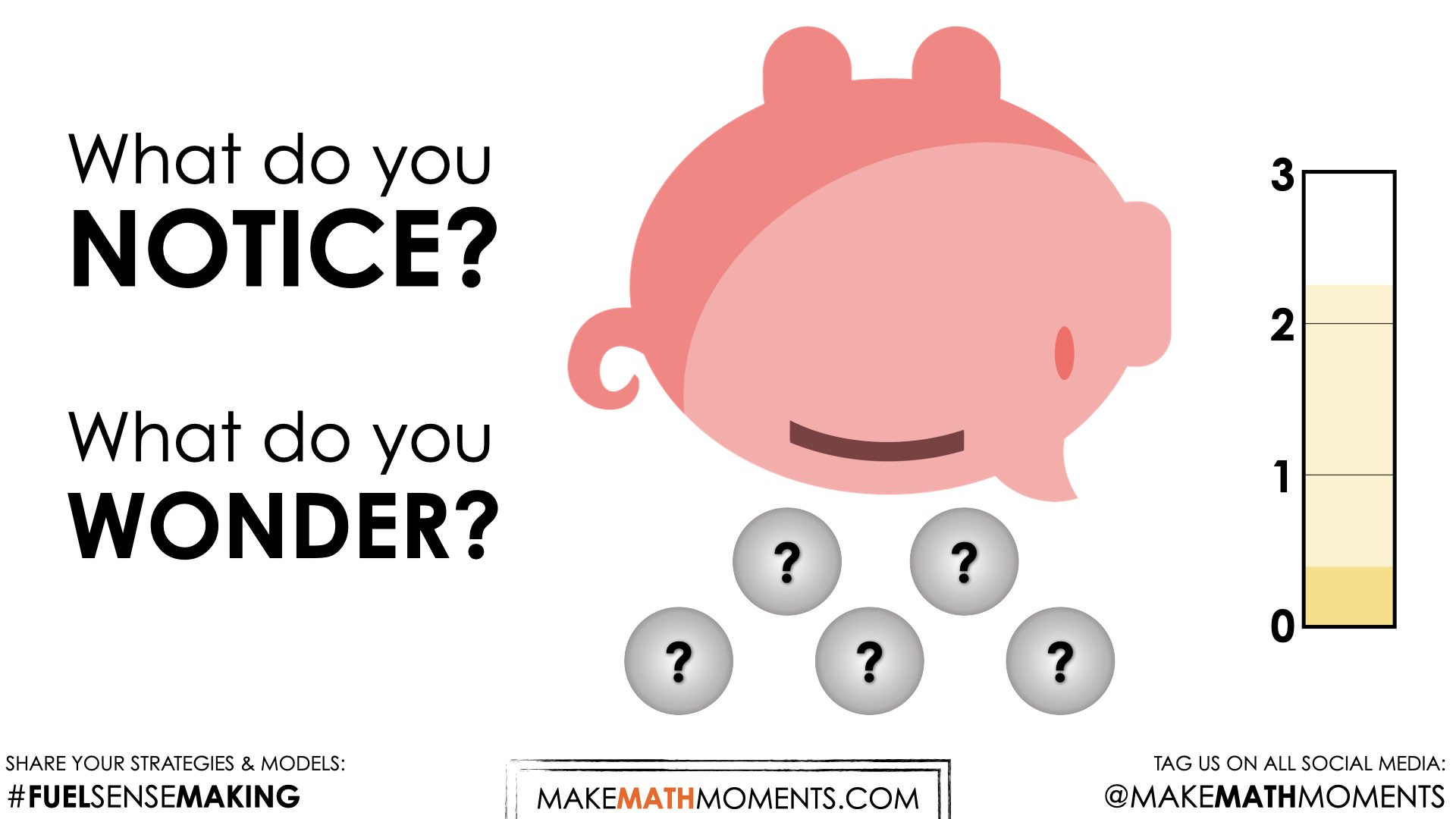Give students 60 seconds (or more) to do a rapid write on a piece of paper.

Then, ask students to share with their neighbours for another 60 seconds.

Finally, allow students to share with the entire group. Be sure to write down these noticings and wonderings on the blackboard/whiteboard, chart paper, or some other means to ensure students know that their voice is acknowledged and appreciated.

Some of the noticing and wondering that may come up includes:

• I notice a piggy bank.
• I notice a bar on the side.
• I notice there was \$2.25 to start.
• I notice that they shook out 5 coins.
• I notice that there is still money left in the bank.
• I wonder what coins they removed.
• I wonder if all of the coins have the same value.
• I wonder how many coins are left.

At this point, you can answer any wonders that you can cross off the list right away. For this particular lesson, you will want to keep most of the information hidden at this time. By sharing too much information, you may rob students of their thinking. One thing you can confirm is that the piggy bank was empty before the first coin was deposited.

## Estimation: Prompt

After we have heard students and demonstrated that we value their voice, we can land on the first questions we will challenge them with:

How much money was in the piggy bank to begin with?

How much money is left in the piggy bank?

Make an estimate.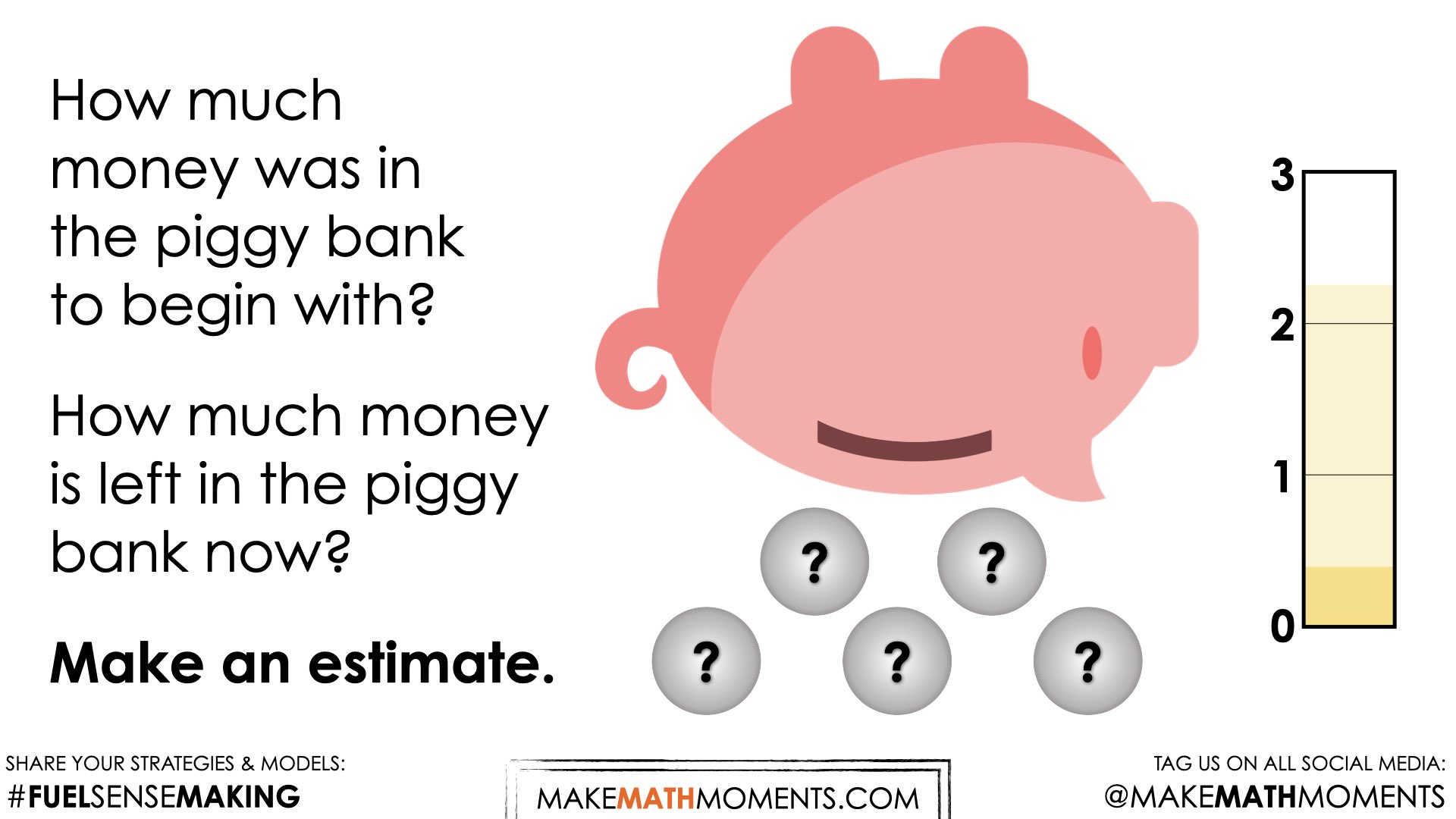We can now ask students to make an estimate (not a guess) as we want them to be as strategic as they can possibly be. This will force them to use spatial reasoning alone to try and come up with an initial estimate and to share it with their neighbours by trying to articulate why they believe their prediction is reasonable.

Consider asking students to think about a value that would be “too low” and a value that would be “too high” before asking for their best estimate in order to help them come up with a more reasonable estimate.

Let them chat with their neighbours and challenge them to an estimation duel or a math fight.

## While Students Are Estimating…

Monitor student thinking by circulating around the room and listening to the mathematical discourse.

Encourage students to use precise mathematical language. You will likely hear students discussing coins using the names of the coins (quarter, dime, nickel), as well as the number of cents. Based on the quantities represented by the metre.

For example, “I think it is less than half a dollar, so it is less than 50 cents”. “I think it is more than one quarter”. “I think they had two dollars and one quarter to start, because it looks like there are four equal parts of that quantity between 2 and 3.”

After students had an opportunity to share their best guess, tell them that you will share some information with them that might help to refine their estimate.

## Crafting A Productive Struggle: Prompt

Show students the following image: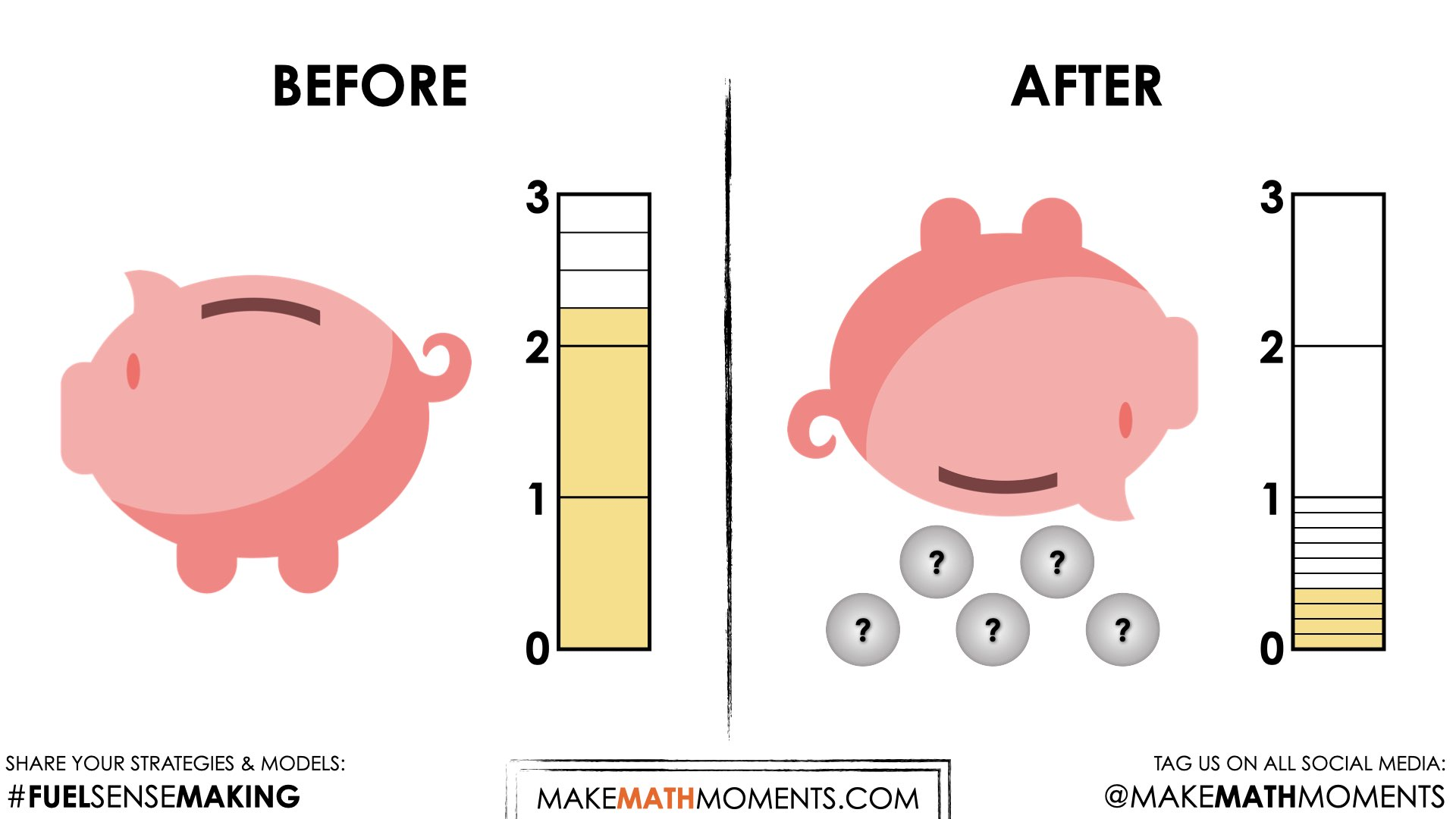At this point you can celebrate the student estimates that were closest to the actual value, 2.25 and 0.40.

Prompt students with:

What five coins were removed from the piggy bank?

Represent this context using a model of your choice and as a single equation.

## While Students Are Productively Struggling…

Monitor student thinking by circulating around the room and listening to the mathematical discourse. Select and sequence some of the student solution strategies and ask a student from the selected groups to share with the class from:

• most accessible to least accessible solution strategies and representations;
• most common/frequent to least common/frequent strategies and representations; or,
• choose another approach to selecting and sequencing student work.

The tools and representations you might see students using to convince their peers and/or the teacher include:

• Coins
• A pictorial representation such as a bar model
• A number line
• Equivalence
• Counting on
• Counting back
• Decomposition
• Difference

Have students share their strategies and reasoning for how to represent each coin that was removed from the piggy bank. Ask them to convince you and their peers that their answer is correct by sharing mathematical models.

Discuss their strategies and elicit student thinking during your consolidation to build off of their current prior knowledge and understanding rather than “fixing” or “funnelling” student thinking to a strategy and/or model that does not connect to their strategy and/or approach.

## Student Approach #1: Digital Fraction Tiles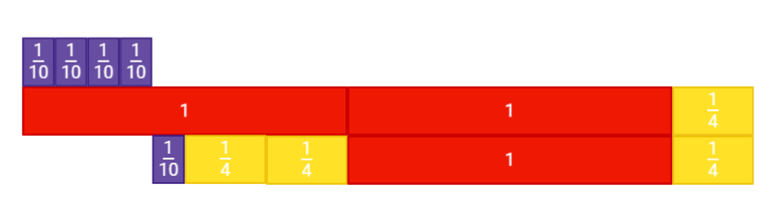I started by representing the amount that was left in the piggy bank with four-tenths, and the amount to start which was 2 dollars and one quarter. Then I used extra tiles to find the difference between the two metres, I knew that I needed only five coins, so I tried different combinations until the pieces fit.

## Student Approach #2: Using a Bar Model to Decompose the Difference Through Counting On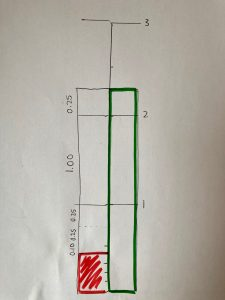I drew the two meters side by side. I knew that the coins removed from the bank are the difference between 0.40 and 2.25. So I started at 0.40, and started making jumps. I added ten cents to get me to 0.50. Then I saw three quarters, and one more loonie. That makes up the five coins removed from the piggy bank.

## Student Approach #3: Open Number Line to Find the Difference and Decompose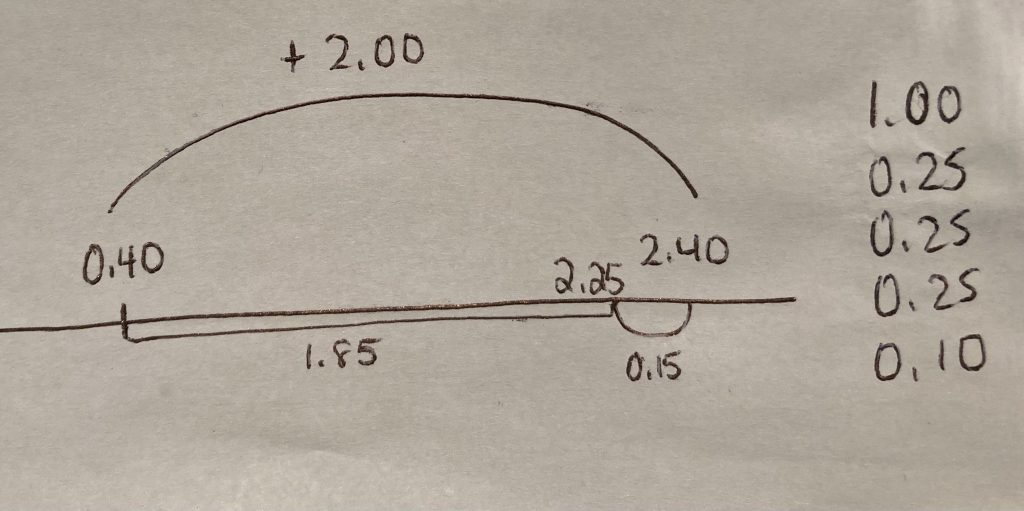I knew that I had to find the difference between the two metres. I started at 0.40 on the number line and I added two dollars. Two dollars was more than \$2.25, so I subtracted \$0.15. That told me that \$1.85 was removed from the piggy bank. Then I just figured out how to use five coins to make that amount. I knew I needed at least one dollar, and then figured out the rest.

## Consolidation

In today’s context, students had the opportunity to connect decimal values to fractional amounts. For example, one quarter (\$2.25) can be represented spatially as two and one-fourth, and \$0.40 can be represented as four tenths (or four dimes).

When asked to represent their thinking, many students may have leveraged a linear bar model similar to the metres used to establish this context. Explore how students went about determining the coin values. It is likely that many students approached this problem by counting on, adding on by taking jumps from 0.40 to 2.25, or decomposing the difference.

Share different student numerical representations of this context. Prompt students by asking:

What operator did we use today?

Many students might indicate that this problem involved addition, as this operator was likely used as a strategy for many learners.

In reality, the context explored today can be represented by a subtraction structure, missing subtrahend. This scenario can be represented as an equation using a variable to represent the unknown quantity (or subtrahend) removed from the piggy bank.

2.25 –= 0.40

Highlight that a variable can be used in an equation to represent an unknown quantity.

Today’s context added an extra level of complexity when we asked students to decompose the subtrahend into five coin values.

You might take this opportunity to share with students that there are in fact four different subtraction structures: removal, comparison, missing subtrahend and missing addend.

It is not necessary to explore all four structures at this time, but students will build flexibility if they come to realize that subtraction can represent more than straightforward removal contexts.

Here is a short silent solution animation that unpacks Student Approach #3 that might be helpful as you prepare for facilitating the consolidation:

## Reflect and Consolidation Prompts

Provide students an opportunity to reflect on their learning by offering these consolidation prompts to be completed independently.

### Consolidation Prompt #1:

Consider the two equations below. Explain how they are connected.

4.10 – ▢ = 2.80

2.80 + 1.30 = 4.10

### Consolidation Prompt #2:

Consider the equation below. Write a context that could be represented by this equation.

3.30 – ▢ = 1.75

We suggest collecting this reflection as an additional opportunity to engage in the formative assessment process to inform next steps for individual students as well as how the whole class will proceed.

## Educator Discussion Area

Login/Join to access the entire Teacher Guide, downloadable slide decks and printable handouts for this lesson and all problem based units.

## Explore Our 60+ Problem Based Units

This Make Math Moments Lesson was designed to spark curiosity for a multi-day unit of study with built in purposeful practice, number talks and extensions to elicit and emerge strategies and mathematical models.

Dig into our other units of study and view by concept continuum, grade or topic!